# Test: Polynomials - 2

## 25 Questions MCQ Test NCERT Mathematics for CAT Preparation | Test: Polynomials - 2

Description
Attempt Test: Polynomials - 2 | 25 questions in 25 minutes | Mock test for Class 9 preparation | Free important questions MCQ to study NCERT Mathematics for CAT Preparation for Class 9 Exam | Download free PDF with solutions
QUESTION: 1

Solution:
QUESTION: 2

Solution:
QUESTION: 3

### A polynomial containing two nonzero terms is called a ________.

Solution:
A binomial is a mathematical expression with two terms.
Examples of binomials.
binomial
All of these examples are binomials. Study them for a bit, and see if you can spot a pattern. The following is a list of what binomials must have:
They must have two terms.
If the variables are the same, then the exponents must be different.
Exponents must be whole positive integers. They cannot be negatives or fractions.
A term is a combination of numbers and variables. In the example 3x + 5, our first term is 3x, and our second term is 5. Terms are separated by either addition or subtraction. In our first example, notice how the 3x and 5 are separated by addition. In the last example, we have a binomial whose two terms both have the same variable s. Notice how each term has its variable to a different exponent. The first term has an exponent of 5, and the second term has an exponent of 4. While we can have fractions for our numbers, we cannot have fractional exponents.
Here are some examples of expressions that are not binomials.
QUESTION: 4

The expanded form of (3x−5)3 is

Solution:

Let a = 3x and b = 5 (3x)³ - (5)³ - 3×3x×5(3x- 5) = 27x³ - 125 -45x ( 3x -5) = 27x³ -125 - 135x² + 225x = 27x³ - 135x² + 225x - 125

QUESTION: 5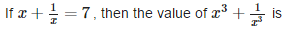Solution:

X+ 1/x = 7 then, cubing both side = (x +1/x)³ = (7)³ = x³ + 1/x³ + 3×X×1/X (x+1/x) = 343 = x³ +1/x³ +3(7) = 343 =x³ +1/x³ +21 =343 = x³ +1/x³ =343 - 21 = x³ +1/x³ = 322

QUESTION: 6

Degree of the polynomial 4x4 + 0x3 + 0x5 + 5x + 7 is:

Solution:

Degree is the highest exponent of any value in an equation. Here, this highest exponent is 4. Therefore, 4 is the degree of the given equation.

QUESTION: 7

(x + 1) is a factor of the polynomial

Solution:
QUESTION: 8

A polynomial containing three nonzero terms is called a ________.

Solution:
QUESTION: 9

If x + 2 is a factor of x3 – 2ax2 + 16, then value of a is

Solution:

use factor theorem as x+2 is factor of
x³-2ax²+16 so put x = -2 and equate the equation to 0
so putting x = -2
(-2)³-2a(-2)²+16 =0
-8-8a+16=0
-8a = -8
a = 8/8= 1
So,
a = 1

QUESTION: 10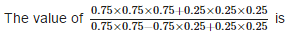Solution:

Solution :- (0.75 * 0.75 * 0.75 + 0.25 * 0.25 * 0.25)/(0.75 * 0.75 - 0.75 * 0.25 + 0.25 * 0.25)

= (0.421875 + 0.015625)/(0.5625 - 0.1875 + 0.0625)

= (0.4375)/(0.4375)

= 1

QUESTION: 11

If one of the factor of x2 + x – 20 is (x + 5). Find the other

Solution:
QUESTION: 12

If 10x−4x2−3, then the value of p(0)+p(1) is

Solution:
QUESTION: 13

The coefficient of x3 in 2x+x2−5x3+x4 is

Solution:
QUESTION: 14

If both x - 2 andare the factors of px2 + 5x + r, then

Solution:

Let f(x) = px2 + 5 x + r
If (x - 2) is a factor of f (x), then by factor theorem
f(2) = 0 | x - 2 = 0 ⇒ x = 2
⇒ p(2)2 + 5(2) + r = 0
⇒ 4p + r + 10 = 0    ...(1)

Ifis a factor of f (x), then by factor theorem,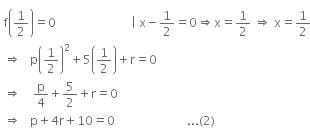Subtracting (2) from (1), we get
3p - 3r = 0
⇒    p = r

QUESTION: 15

If x + 2 is a factor of x3 – 2ax2 + 16, then value of a is

Solution:
QUESTION: 16

The value of the polynomial 5x−4x2+35, when x = −1 is

Solution:
QUESTION: 17

If x2+kx+6 = (x+2)(x+3), then the value of ‘k’ is

Solution:
QUESTION: 18

The remainder when the polynomial x4+2x3−3x2+x−1 is divided by (x−2) is

Solution:
QUESTION: 19

The remainder obtained when the polynomial p(x) is divided by (b – ax) is

Solution:

Solution :- b-ax=0

b=ax

b/a=x

i.e. remainder is p(b/a)

QUESTION: 20

The value of x3+y3+15xy−125 when x+y = 5 is

Solution:

x³+y³+15xy-125
=x³ + y³ +3 xy ×5 - 125
=x³ + y³ +3xy(x+y) - (5)³
= (x+y) ³ - (5)³
=(5)³ - (5)³
=0

QUESTION: 21

If p(x) = x + 3, then p(x) + p(-x) is equal to

Solution:
Given p(x) = x+3, put x = -x in the given equation, we get p(-x) = -x+3
Now, p(x)+ p(-x) = x+ 3+ (-x)+ 3=6
QUESTION: 22

One of the factors of (16y2−1)+(1−4y)2 is

Solution: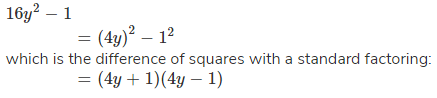QUESTION: 23

If the polynomial x3−6x2+ax+3 leaves a remainder 7 when divided by (x−1), then the value of ‘a’ is

Solution:
QUESTION: 24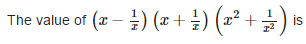Solution:
QUESTION: 25

The value of (a2−b2)3+(b2−c2)3+(c2−a2)3 is

Solution: Let (a²-b²) =x , (b²-c²) =y , (c²-a²) = z we know that a+b+ c=0 and, a³ + b³ + c³ = 3abc so, 3(a+b)(a-b)(b+c) (b-c)(c+a)(c-a) = 3(a+b)(b+c)(c+a)(a-b)(b-c)(c-a)Use Code STAYHOME200 and get INR 200 additional OFF Use Coupon Code